# 10.3 SPM Practice (Long Questions)

Question 4: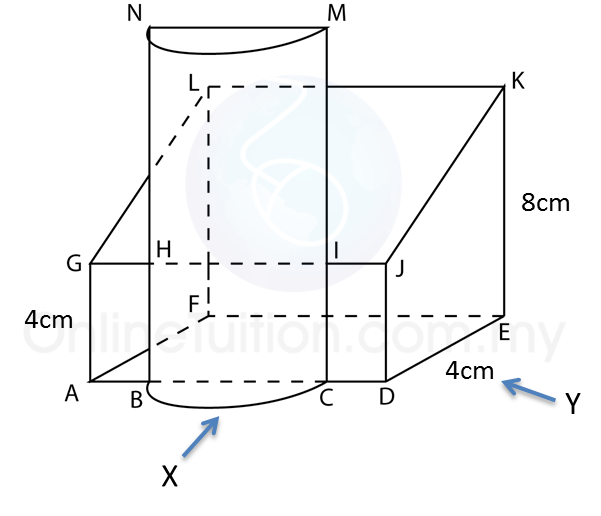The diagram above shows a solid consisting of a right prism and a half-cylinder which are joined at the plane HICB. The base ABCDEF is on a horizontal plane. The rectangle LKJG is an inclined plane. The vertical plane JDEK is the uniform cross-section of the prism. AB = CD = 2 cm. BC = 4 cm. CM = 12 cm.
Draw to full scale
(a)  The plan of the solid
(b) The elevation of the solid on a vertical plane parallel to ABCD as viewed from X.
(c)  The elevation of the solid on a vertical plane parallel to DE as viewed from Y.

Solution:
(a)(b)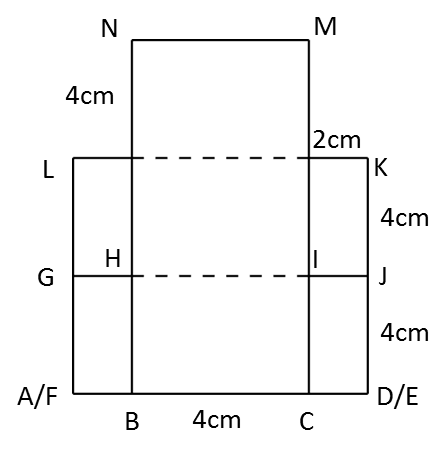(c)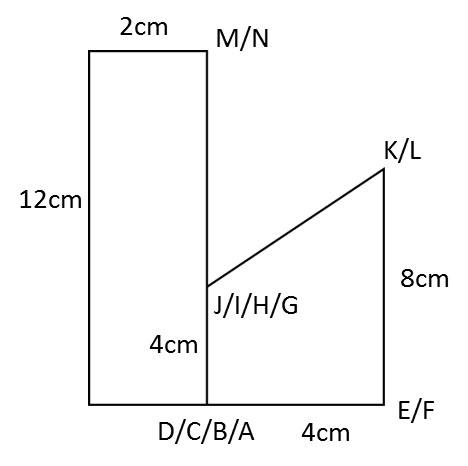# 10.3 SPM Practice (Long Questions)

Question 1: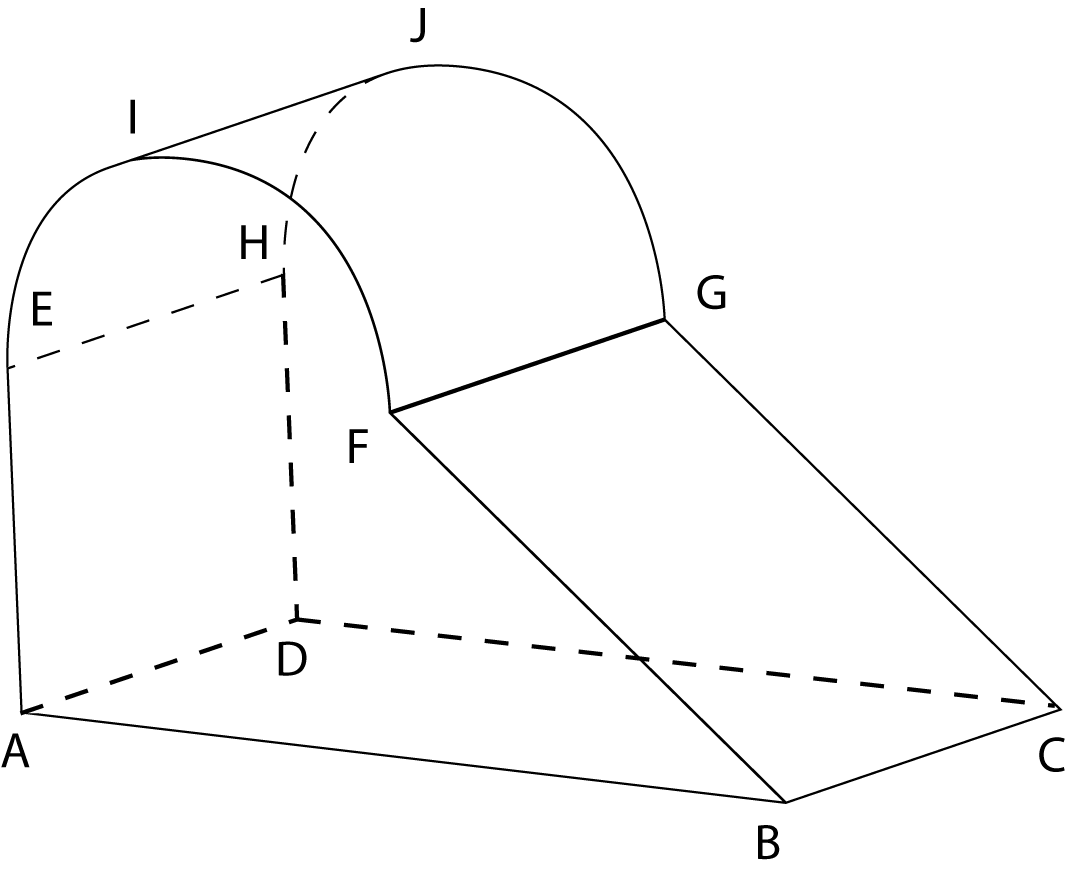The diagram above shows a solid consisting of a right prism and a half-cylinder which are joined at the plane EFGH. EF is the diameter of the semi-circle and is equal to 3 cm.

The base ABCD is on a horizontal plane and AB = 6 cm, BC = 4 cm. The vertical plane ABFE is the uniform cross-section of the prism.

Draw to full scale, the plan of the solid.

Solution:Question 2:Diagram above shows a solid right prism with rectangular base ABCD on a horizontal table. The vertical plane ABEHIL is the uniform cross-section of the prism. Rectangle LIJK, IHGJ and HEFG are inclined planes. AL, DK, BE and CF are vertical edges.

Given BC
= 4 cm, AB = 6cm. EB = FC = LA = KD = 4cm, The vertical height of  I and J from the rectangular base ABCD = 3cm, while the vertical height of H and G from the rectangular base ABCD = 5cm.

Draw to full scale, the elevation of the solid on a vertical plane parallel to BC as viewed from X.

Solution: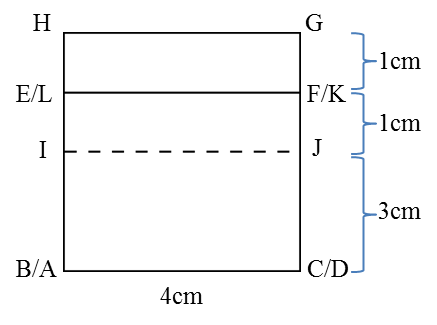# 10.2 Plans and Elevations

10.2 Plans and Elevations

1.  Plan is the image formed when solid is viewed from the top. Its orthogonal projection lies on the horizontal plane.
2.  Elevation is the image formed when a solid is viewed from the front or from the side. Its orthogonal projection lies on the vertical plane.
3.  In drawing plans and elevations of solids,
(a)  Visible edges should be drawn using solid lines(──),
(b)  Hidden edges whose views are blocked should be drawn using dashed lines (- - -).

Example:# 10.3 SPM Practice (Long Questions)

Question 3:The diagram above shows a right prism attach to a cuboid at one of its plane. ABCG and CDEF, IJNH and KLMJ are horizontal planes, ABIH, AGNH and GCJN are vertical planes while IBCJ is an inclined plane. HA = 3 cm, BC = 3 cm, CD = 1.5 cm, HI = NJ = JK = 3 cm. Draw to full scale

(a) The elevation of the solid on a vertical plane parallel to AB as viewed from X.(b) A solid semi-cylinder is joined to the solid in A as show in the diagram above. JPON is a vertical plane and JP = 1.5 cm. Draw to full scale
i. The plan of the solid
ii.   The elevation of the solid on a vertical plane parallel to BCD as viewed from Y.

Solution:

(a)(b)(i)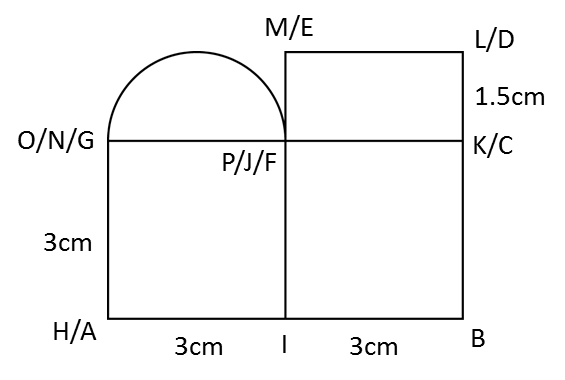(b)(ii)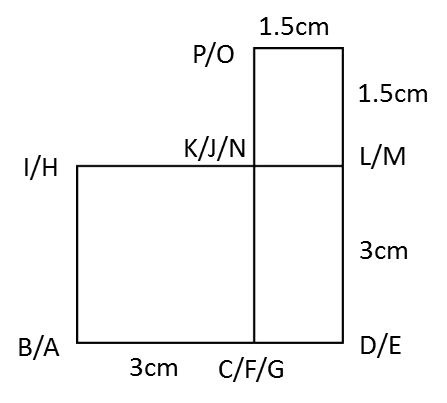# 9.4.3 Shortest Distance between Two Points

9.4.3 Shortest Distance between Two Points

1. The shortest distance between two points on the surface of the earth is the distance measured along a great circle.Shortest distance between points D and M    = ( θ × 60 ) nautical miles

Example: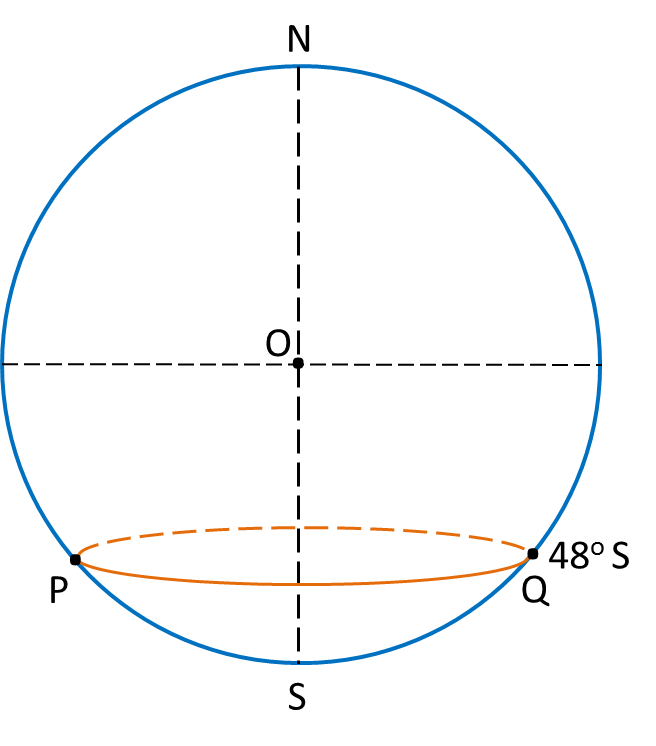In the above diagram, calculate
(a) The distance from P to Q, measured along the parallel of latitude 48o S,
(b) The distance from P to Q, measured along the route PSQ, where S is the South Pole.
State the shorter distance.

Solution: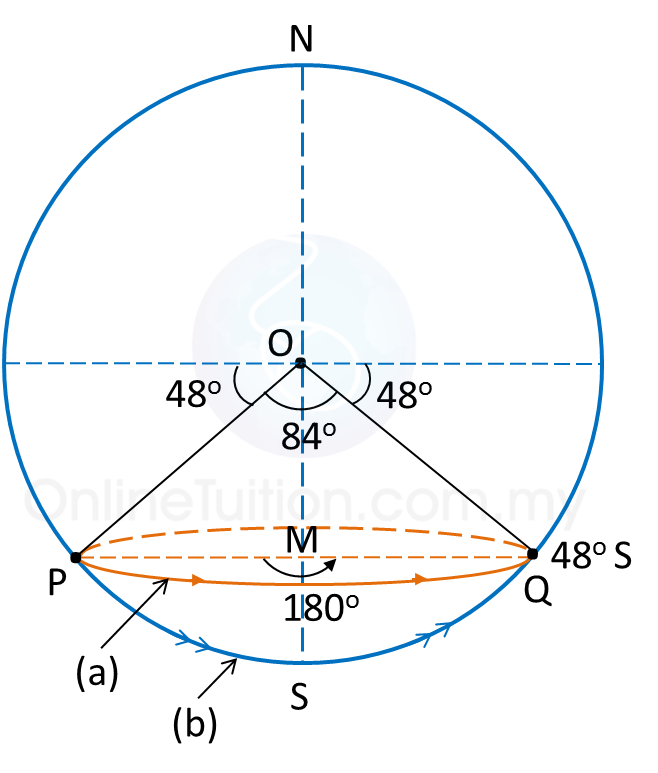(a)
Distance from Pto Q, measured along the parallel of latitude 48o S
= 180 × 60 × cos 48o← (angle PMQ = 180o)
= 7266.61 n.m.

(b)
Distance from Pto Q, measured along the route PSQ, where S is the South Pole
= 84 × 60 ← (angle POQ = 180o – 48o – 48o = 84o)
= 5040 n.m.

The distance from P to Q, measured along the route PSQ in (b), where S is the South Pole, is shorter than the distance measured along the parallel of latitude in (a).

 The shortest distance in the above example is       the distance along the arc of a great circle,      which passes through the South (or North) Pole.

# 9.3 Location of a Place

9.3 Location of a Place

The location of a place is written as an ordered pair of latitude and longitude (latitude, longitude).

Example:Location of point P is (35o N, 27o E).

# 9.6 SPM Practice (Long Questions)

Question 1:
Diagram below shows four points P, Q, R and M, on the surface of the earth. lies on longitude of 70oW. QR is the diameter of the parallel of latitude of 40o N. M lies 5700 nautical miles due south of P.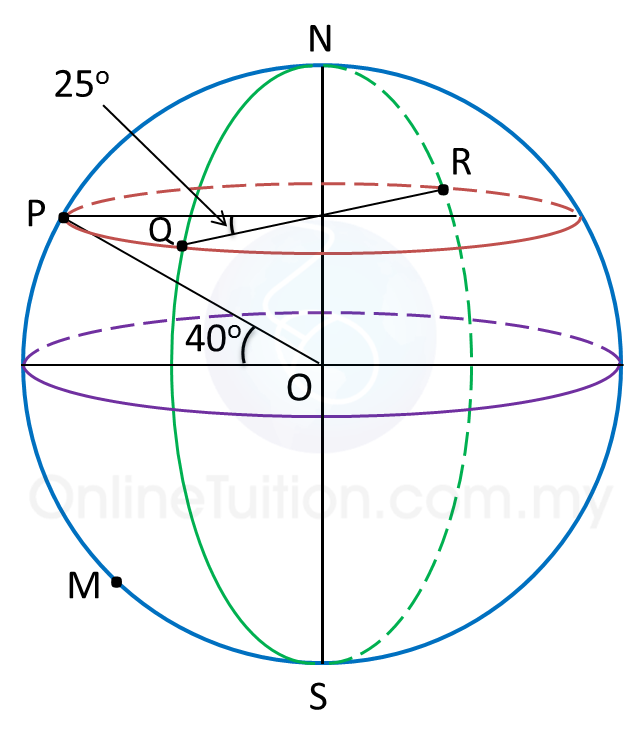(a) Find the position of R.

(b)
Calculate the shortest distance, in nautical miles, from Q to R, measured along the surface of the earth.

(c)
Find the latitude of M.

(d)
An aeroplane took off from R and flew due west to P along the parallel of latitude with an average speed of 660 knots.

Calculate the time, in hours, taken for the flight.

Solution:
(a)
Latitude of = latitude of Q = 40o N
Longitude of = (70o – 25o) W = 45o W
Longitude of = (180o – 45o) E = 135o E
Therefore, position of R = (40o N, 135oE).

(b)
Shortest distance from Q to R
= (180 – 40 – 40) x 60
= 100 × 60
= 6000 nautical miles

(c)
$\begin{array}{l}\angle POM=\frac{5700}{60}\\ \text{}={95}^{o}\\ \therefore \text{Latitude of}M=\left({95}^{o}-{40}^{o}\right)S\\ \text{}={55}^{o}S\end{array}$

(d)
$\begin{array}{l}\text{Time taken =}\frac{\text{distance from}R\text{to}P}{\text{average speed}}\\ \text{}=\frac{\left(180-25\right)×60×\mathrm{cos}{40}^{o}}{660}\\ \text{}=\frac{155×60×\mathrm{cos}{40}^{o}}{660}\\ \text{}=10.79\text{hours}\end{array}$

# 9.6 SPM Practice (Long Questions)

Question 2:
P(25o S, 40o E), Qo N, 40o E), R(25o S, 10o W) and are four points on the surface of the earth. PK is the diameter of the earth.

(a)
State the location of point K.

(b)
Q is 2220 nautical miles from P, measured along the same meridian.
Calculate the value of θ.

(c)
Calculate the distance, in nautical mile, from P due west to R, measured along the common parallel of latitude.

(d)
An aeroplane took off from Q and flew due south to P. Then, it flew due west to R. The average speed of the aeroplane was 600 knots.
Calculate the total time, in hours, taken for the whole flight.

Solution:(a)
As PK is the diameter of the earth, therefore latitude of K = 25o N
Longitude of K= (180o – 40o) W = 140o W
Therefore, location of K = (25o N, 140oW).

(b)
Let the centre of the earth be O.
$\begin{array}{l}\angle POQ=\frac{2220}{60}\\ \text{}={37}^{o}\\ {\theta }^{o}={37}^{o}-{25}^{o}={12}^{o}\\ \therefore \text{The value of}\theta \text{is 12}\text{.}\end{array}$

(c)
Distance from to R
= (40 + 10) × 60 × cos 25o
= 50 × 60 × cos 25o
= 2718.92 n.m.

(d)
Total distance travelled
= distance from to P + distance from P to R
= 2220 + 2718.92
= 4938.92 nautical miles
$\begin{array}{l}\text{Time taken =}\frac{\text{total distance from}Q\text{to}R}{\text{average speed}}\\ \text{}=\frac{4938.92}{600}\\ \text{}=8.23\text{hours}\end{array}$

# 9.5 SPM Practice (Short Questions)

Question 1
In diagram below, N is the North Pole and is the South Pole. The location of point is (40o S, 70o W) and POQ is the diameter of the earth.Find the longitude of Q.

Solution:
Since PQ is a diameter of the earth and the longitude of P is θo W, the longitude of Q is (180o – θo) E.
Longitude of = 70o W
Longitude of = (180o – 70o) E
= 110oE

Question 2
In diagram below, N is the North Pole and is the South Pole and NOS is the axis of the earth.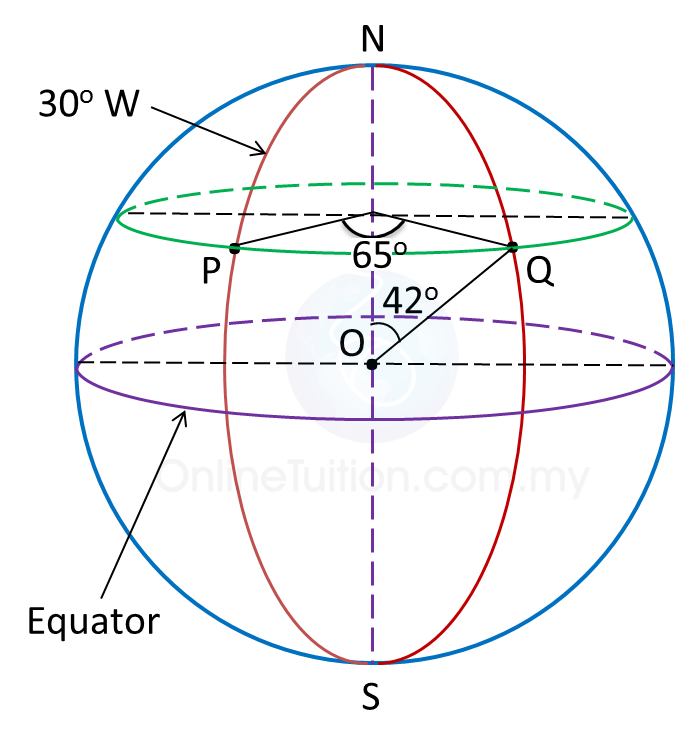Find the position of point Q.

Solution:
Latitude of = (90o – 42o) N
= 48o N
Longitude of = (65o – 30o) E
= 35o E
Therefore, position of Q = (48o N, 35oE).

# 9.1 Longitudes

9.1 Longitudes

1. A great circle is a circle with the centre of the Earth as its centre.
2. A meridian is half of a great circle from the North pole to the South pole.
3. The longitude of the Greenwich Meridian is 0o.
4. The longitude of a meridian is determined by:
(a) The angle between the meridian plane and the Greenwich Meridian.
(b) The position of the meridian to the east or west of the Greenwich Meridian.

Example:Longitude of is 55o W.
Longitude of is 30o E.

5. All points on the same meridian have the same longitude.

Difference between Two Longitudes
1. If both the meridians are on the east (or west) of the Greenwich Meridian, subtract the angles of the longitudes.
2. If both the meridians are on the opposite sides of the Greenwich Meridian, add the angles of the longitudes.